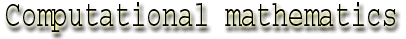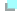aaaaaaaaaaaaaaaaaShannon's Sampling Theorem aaaaaaaaaaaaaaaaaThe concept of entropy aaaaaaaaaaaaaaaaaUseful links Back to table of contents According to Aaron D. Wyner, another mathematician that worked at Bell Labs, "Information theory has had an important and significant influence on mathematics, particularly on probability theory...In the course of developing the solutions to the basic communication problem, Shannon introduced several mathematical concepts. Primary among these is the notion of the "entropy" of a random variable (and by extension of a random sequence), the "mutual information" between two random variables or sequences, and a calculus that relates these quantities and their derivatives."Shannon's Sampling Theorem Shannon came up with the sampling theorem when he needed to find a solution to the problem of how computers can handle a continuous analogue signal. He concluded that this could be done by sampling, i.e. recording the value of the continuous signal at regular points to get a sequence of numbers. Enough information is needed to reconstruct the signal but too much information will be unmanageable by the computer. Shannon realized that the sampling frequency has to be at least twice the absolute maximum frequency of the continuous signal. This sampling frequency is also called the Nyquist frequency. A formal definition of the theorem is as follows: In order for a band-limited (i.e. one with a zero Power Spectrum for frequencies f>B) baseband (f>0) signal to be reconstructed fully, it must be sampled at a rate f>=2B. A signal sampled at f=2B is said to be Nyquist Sampled, and f=2B is called the Nyquist Frequency. No information is lost if a signal is sampled at the Nyquist Frequency, and no additional information is gained by sampling faster than this rate. Definitions Power Spectrum - For a given signal, the power spectrum gives a plot of the portion of a signal's power (energy per unit time) falling within given frequency bins. Nyquist Frequency - In order to recover all components of a periodic waveform, it is necessary to sample twice as fast as the highest waveform frequency v. FNyquist=2v. The minimum sampling frequency is called the Nyquist frequency. Back to table of contents Top of pageThe concept of entropyEntropy in logic and theory of algorithms The idea of a random number existed for a long time in mathematics. However, recognizing a random number rested mainly on intuition. Clearly a more sensible definition of randomness was required. The first mathematical definition was provided by Shannon who actually came up with a formula that defined entropy (the degree of randomness in a system). Other definitions followed but they all have their heritage in information theory. Some examples of mathematicians that developed the idea of entropy are Ray Solomonoff who devised a notion of algorithmic probability and Martin-Loef who used similar ideas to develop a theory of algorithmic randomness. Also, in 1965, A. N. Kolmogorov of the Academy of Science of the U.S.S.R. and Gregory Chaitin, then an undergraduate at the City College of the City University of New York. proposed indepedently their own algorithmic definition of randomness. A brief explanation of the algorithmic definition of randomness (based on Chaitin's definition as found on his on-line article: A series of numbers is random if the smallest algorithm capable of specifying it to a computer has about the same number of bits of information as the series itself. Any minimal program is necessarily random, whether or not the series it generates is random. This conclusion is a direct result of the way randomness is defined. Consider the program P, which is a minimal program for the series of digits S. Assuming that P is not random, by definition there must be another program, P', smaller than P, that will generate it. S can then be produced by the following algorithm: From P' calculate P, then from P calculate S This program is only a few bits longer than P', and thus it must be substantially shorter than P. P is therefore not a minimal program. According to Chaitin, the algorithmic definition of randomness provides a new foundation for the theory of probability. It does not supersede classical probability theory, which is based on an ensemble of possibilities, each with an assigned probability. Instead, it complements the ensemble method by giving precise meaning to concepts that were mainly intuitive. Chaitin's work however, concentrates mostly on the limitations of this definition. The definition cannot help to determine whether or not a given series of digits is in fact random or only seems to be random. According to Chaitin, this limitation is not a flaw in the definition but a consequence of a subtle but fundamental anomaly in the foundation of mathematics. It is closely related to a famous theorem devised and proved in 1931 by Kurt Gödel, which has come to be known as Gödel's incompleteness theorem. In addition to the algorithmic definition, Chaitin, using Shannon's entropy, related the minimal program to the concept of complexity.The complexity of a series of digits is the number of bits that must be put into a computer in order to get the original series as output. Thus, the complexity is equal to the size in bits of the minimal programs of the series. This gives another definition of randomness: A random series of digits is one whose complexity is approximately equal to its size in bits. The notion of complexity serves to measure randomness. Given many series of numbers each with n digits, it is theoretically possible to identify all those of complexity n-1, n-10, n-100 etc, and to rank the series in decreasing order of randomness. The complexity of a random series is approximately equal to the size of the series. The methods of the algorithmic theory of probability, that have their roots in Shannon's work, reveal many of the properties of both random and nonrandom numbers. According to Chaitin, the frequency distribution of digits in a series has an important influence on the randomness of the series. For instance, a series consisting entirely of either 0's or 1's is far from random - the algorithmic approach confirms that conclusion. If such a series is n digits long, its complexity is almost equal to log2n. The series can be produced by a simple algorithm such as: Print 0 n times. in which the information needed is contained in the binary numeral for n, i.e. log2 n bits. Since, even for a long series, the logarithm of n is much smaller than n itself, such numbers are of low complexity. Thus, to demonstrate that a particular series of digits is random, you must prove that no small program for calculating it exists.Entropy in statistical inference and prediction Examples of people that used Shannon's theory in this field: Ronald A. Fisher introduced a notion of information into the theory of statistical inference in 1925. This Fisher information is now understood to be closely related to Shannon's notion of entropy. In 1951, Solomon Kullback introduced the divergence between two random variables (this quantity is also called the cross entropy, discrimination, Kullback-Liebler entropy, etc.) and found another connection between Shannon's entropy and the theory of statistical inference. Meir Feder, Neri Merhav and Michael Gutman wrote in 1992 the book Universal Prediction of Individual Sequences, Their starting point in writing this paper was the observation that Shannon's intuition that the entropy of an information source measures how well its behavior can be predicted, together with the existence of Universal encoders (e.g. the Lempel-Ziv algorithm), suggests that there should be an algorithm for predicting the next symbol in a sequence which is guaranteed to become as accurate as desired. Back to table of contents Top of pageUseful links Back to table of contents Top of page